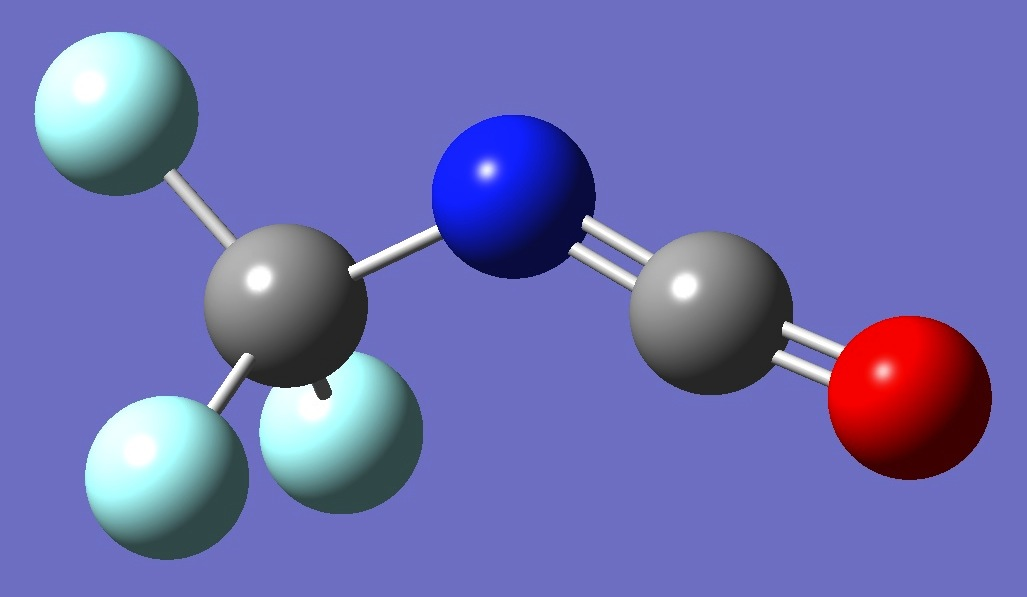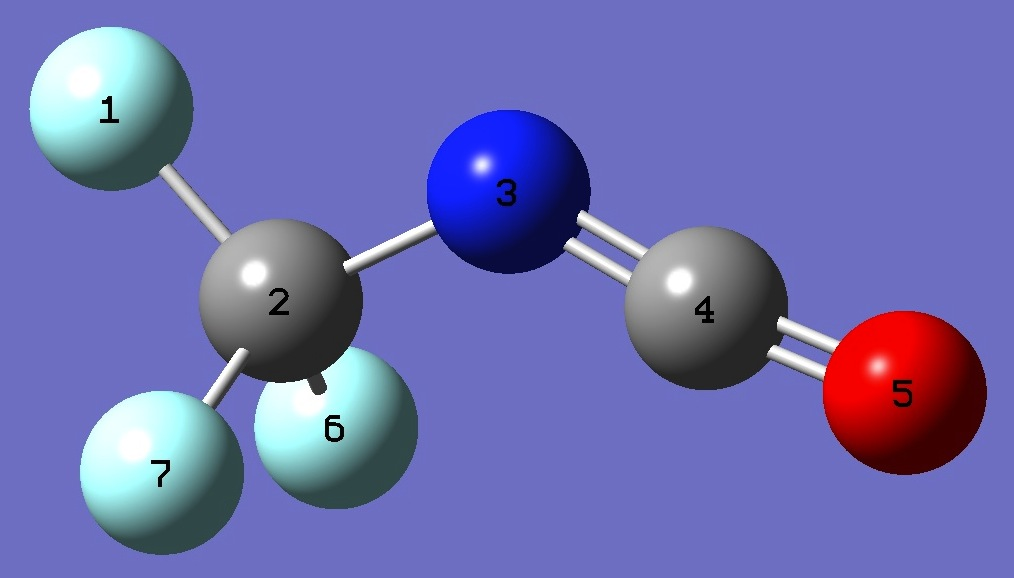CF3N=C=ONitrogen

Nuclear Quadrupole Coupling Constants

in Trifluoromethyl Isocyanate

Calculation was made of the 14N nqcc tensor in trifluoromethyl isocyanate on ropt structures given by B3LYP/6-311+G(3df,3pd) and MP2/6-311+G(3df,3pd) optimization.

In Table 1, these calculated nqcc's are compared with the experimental values of Koput et al. .  Subscripts a,b,c refer to the principal axes of the inertia tensor.  Subscripts x,y,z refer to the principal axes of the nqcc tensor.  ETA = (Xxx - Xyy)/Xzz.  Ø (degrees) is the angle between its subscripted parameters.  RMS is the root mean square difference between calculated and experimental nqcc's (percentage of average experimental nqcc).  RSD is the residual standard deviation of calibration of the B3PW91/6-311+G(df,pd) model for calculation of the efg's/nqcc's.

Structure parameters are given in Z-matrix format in Table 2, rotational constants are given in Table 3.

 Table 1.  14N nqcc's in CF3N=C=O (MHz).  Calculation was made on ropt molecular structures given by B3LYP/6-311+G(3df,3pd) and MP2/6-311+G(3df,3pd) optimization.  NOTE: Calculated Xbb and Xcc have been reversed (see below). Calc. B3LYP Calc. MP2 Expt.  Xaa 3.309 3.249 3.2977(8) Xbb - 2.079 - 2.118 - 2.1313(40) Xcc - 1.230 - 1.132 - 1.1664(40) |Xac| 0.258 0.209 0.227(19) RMS 0.048 (2.2 %) 0.035 (1.6 %) RSD 0.030 (1.3 %) 0.030 (1.3 %) Xxx - 2.079 - 2.118 - 2.1313 Xyy - 1.245 - 1.142 - 1.1779 Xzz 3.324 3.259 3.3092 ETA 0.251 0.299 Øz,a 3.25 2.73 2.90 Øa,N=C Øz,N=C

 Table 2.  CF3N=C=O.  Structure parameters, B3LYP/6-311+G(3df,3pd) and MP2/6-311+G(3df,3pd) (Å and degrees).F  C,1,B1  N,2,B2,1,A1  C,3,B3,2,A2,1,D1,0  O,4,B4,3,A3,2,D2,0  F,2,B5,3,A4,4,D3,0  F,2,B6,3,A5,4,D4,0 B3LYP MP2 B1=1.33036372  B2=1.40287148  B3=1.21304848  B4=1.15563981  B5=1.34339482  B6=1.34339482  A1=109.56903999  A2=130.97237598  A3=173.3896775  A4=111.94970035  A5=111.94970035  D1=180.  D2=180.  D3=-60.23444432  D4=60.23444432 B1=1.32292923  B2=1.40462186  B3=1.22216511  B4=1.16338695  B5=1.33485641  B6=1.33485641  A1=109.21817901  A2=127.75175369  A3=172.70146532  A4=111.76568662  A5=111.76568662  D1=180.  D2=180.  D3=-60.27908605  D4=60.27908605

NOTE:  As can be seen in Table 1, good agreement between calculated and experimental inertial axes nqcc's is obtained if calculated Xbb and Xcc are reversed.  As can be seen in Table 3, there is little difference between B and C rotational constants.  Calculated rotational constants are rigid molecule values, whereas the experimental values are measured in the ground vibrational state of the molecule.  So, I assume that consideration of zero point vibrational effects could easily reverse the nearly equal Be and Ce, and thus Xbb and Xcc.  Alternatively, small changes in some optimized structural parameters could accomplish this same reversal.

 Table 3.  CF3N=C=O.  Rotational Constants (MHz). B3LYP MP2 Expt  Ae 5637 5685 Ao 5675 (fixed) Be 1719 1744 Bo 1752.5237(20) Ce 1715 1739 Co 1746.1322(20)

 J.Koput, W.Stahl, N.Heineking, G.Pawelke, B.Steger, and D.Christen, J.Mol.Spectrosc. 168,323(1994).

HNCO
CH2C(H)NCO (CH3)3CNCO
CH3CH2NCO

CH3NCO

Molecules/Nitrogen

CF3NCO.html